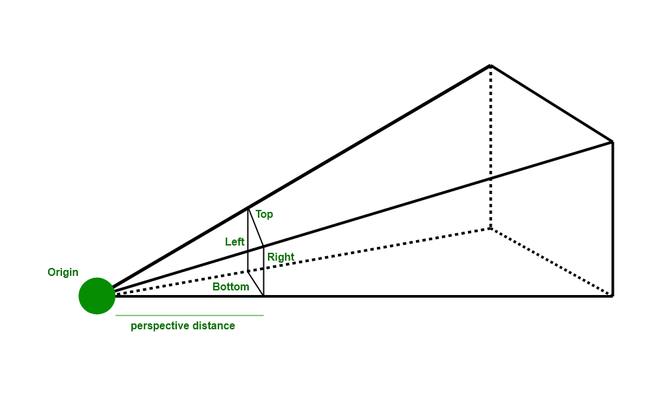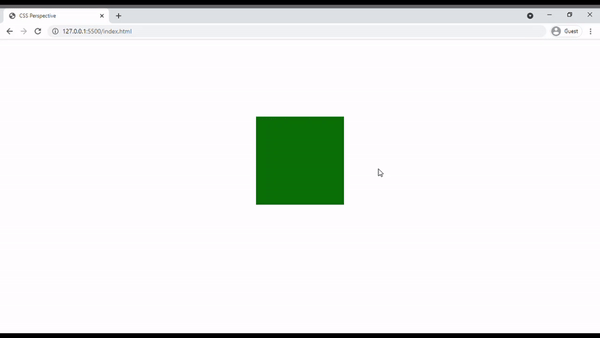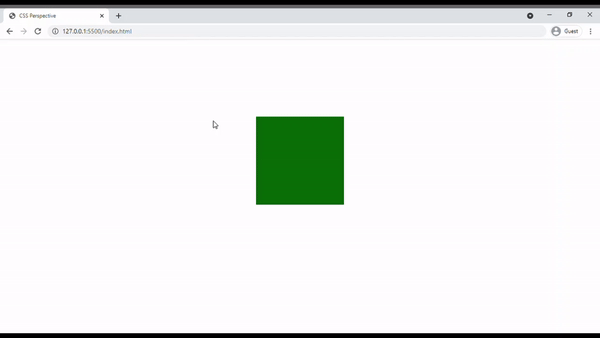# How to set the perspective from where an element is viewed in CSS ?

In this article we will see, how the CSS perspective works. The CSS perspective is a new CSS property that has to provide to manage the distance between the z-axis. We all know that we work with a 2D surface. So if we rotate our objects, then this completeness is not displayed. The solution to this problem is the CSS perspective which we see even with the z-axis.They work like a projector if the source is far from the object so making a big image on the wall. They are used very simply by applying the perspective property to the parent element and make any transformation in the child element that looks like a 3D element.

Syntax:

```/* parent div */
perspective: perspective_distance;

/* child div */
transform: value;```

If the parent div applied perspective so any property of transform in the child apply so they behave as a 3D object

Example 1: Below example is illustrated without using Perspective.

## HTML

 `` `<``html` `lang``=``"en"``>` `<``head``>` `  ``<``style``>` `    ``body {` `        ``display: flex;` `        ``justify-content: center;` `        ``align-items: center;` `        ``height: 80vh;` `     ``}`   `    ``/* parent div */` `    ``.main{` `        ``width: 200px;` `        ``height: 200px;` `        ``background-color: gray;` `     ``}`   `    ``/* child div */` `    ``.box {` `        ``width: 200px;` `        ``height: 200px;` `        ``background-color: #0B7008;` `        ``/* transform the child object. */` `        ``transform: rotateX(60deg);` `     ``}` `  ``` ``   `<``body``>` `` `    ``<``div` `class``=``"main"``>` `` `        ``<``div` `class``=``"box"``>` `    ``` ``   ``

Output:Example 2: Below is the example that illustrates the use of Perspective.

## HTML

 `` `<``html` `lang``=``"en"``>`   `<``head``>` `  ``<``style``>` `      ``body {` `          ``display: flex;` `          ``justify-content: center;` `          ``align-items: center;` `          ``height: 80vh;` `      ``}`   `      ``/* parent div */` `      ``.main{` `          ``width: 200px;` `          ``height: 200px;` `          ``background-color: gray;` `          ``/* Create perspective */` `          ``perspective: 800px;` `      ``}`   `      ``/* child div */` `      ``.box {` `          ``width: 200px;` `          ``height: 200px;` `          ``background-color: #0B7008;` `          ``/* transform the child object. */` `          ``transform: rotateX(60deg);` `      ``}` `  ``` ``   `<``body``>` `` `    ``<``div` `class``=``"main"``>` `` `      ``<``div` `class``=``"box"``>` `    ``` ``   ``

Output:Supported Browser: